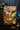# Big O Notation

Allows us to express how the runtime of an algorithm grows as the inputs grow.

## O(1)

``````function addUpTo(n) {
return n * (n + 1) / 2;
}``````
`addUpToN()` performs three operations each time it is called.
1 addition, 1 multiplication, and 1 division.
However, the number of operations does NOT increase with the size of the argument. In general the complexity is O(1)

# O(n)

``````function addUpTo(n) {
let total = 0;
for (let i = 0; i <= n ; i++) {
total += i;
}
}``````
In contrast, the second version is much less efficient. The number of operations grows as n grows. In the for loop, we compare i to n, n times, total += i, n times, i++ n times. It adds up. I general, we say the complexity is O(N).

# O(n^2)

``````function nestedForLoop(n) {
for (var i=0; i < n; i++) {
for (var j=0; j < n; j++) {
console.log(i, j);
}
}
}``````
Here, we have an O(n) operation inside of an O(n) operation, which results in an O(n^2) complexity.

## Some shortcuts

1. Arithmetic operations are constant.

2. Variable assignment is constant.

3. Accessing elements in an array is constant.

4. in a loop, the complexity is the length of the loop times the complexity of what happens inside the loop.